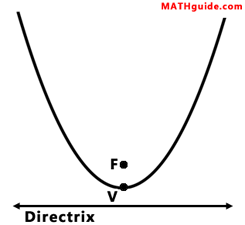Equations of Parabolas
View the Lesson | MATHguide homepage Updated July 7th, 2021

 Given: A parabola with the focus F(9, -7) and directrix y = -15.Calculate: The location of the vertex and the equation of the parabola.V(, )(x - + )2 = (y - + )